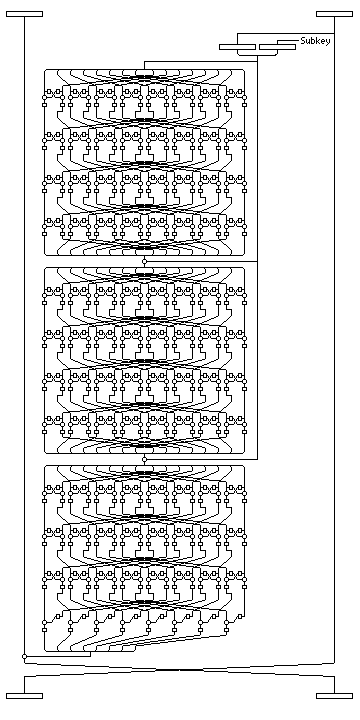[Next] [Up] [Previous] [Index]

Magenta

Magenta is a block cipher with a complex and deeply nested design. However, the S-box has a simple structure, and there are also weaknesses in the key schedule. This led to cryptanalytic results being obtained against it shortly after it was disclosed. Although it has been claimed that there are other weaknesses in the design, it still seems to me that the design contains some useful principles.

The S-box used in Magenta is the following:

1     2     4     8    16    32    64   128   101
202   241   135   107   214   201   247   139   115
230   169    55   110   220   221   223   219   211
195   227   163    35    70   140   125   250   145
71   142   121   242   129   103   206   249   151
75   150    73   146    65   130    97   194   225
167    43    86   172    61   122   244   141   127
254   153    87   174    57   114   228   173    63
126   252   157    95   190    25    50   100   200
245   143   123   246   137   119   238   185    23
46    92   184    21    42    84   168    53   106
212   205   255   155    83   166    41    82   164
45    90   180    13    26    52   104   208   197
239   187    19    38    76   152    85   170    49
98   196   237   191    27    54   108   216   213
207   251   147    67   134   105   210   193   231
171    51   102   204   253   159    91   182     9
18    36    72   144    69   138   113   226   161
39    78   156    93   186    17    34    68   136
117   234   177     7    14    28    56   112   224
165    47    94   188    29    58   116   232   181
15    30    60   120   240   133   111   222   217
215   203   243   131    99   198   233   183    11
22    44    88   176     5    10    20    40    80
160    37    74   148    77   154    81   162    33
66   132   109   218   209   199   235   179     3
6    12    24    48    96   192   229   175    59
118   236   189    31    62   124   248   149    79
158    89   178     0

Start with 1, and shift one position left to obtain the next entry: when 1 is shifted out, XOR the contents with 101. This obtains the first 255 entries of the table; use 0 as the last entry.

In the Magenta documentation, f(x) is defined as entry x in this S-box. On that basis, other functions are defined in a step by step manner:

A(x,y) = f(x xor f(y)) [x and y are both bytes]

PE(x,y) = (A(x,y),A(y,x)) [that is, PE(x,y) is A(x,y) catenated with A(y,x)]

pi(x(0), x(1), ... x(15)) = ( PE(x(0),x(8)), PE(x(1),x(9)), PE(x(2),x(10)), ... PE(x(7),x(15)) )

To help keep track of where we are so far, this diagram illustrates how pi(X) operates on a 16-byte bit string:T(w) = pi(pi(pi(pi(w)))) [where w is a 16-byte vector]

S(x(0),x(1),x(2),...x(15)) = (x(0),x(2),x(4),...x(14),x(1),x(3),x(5),...x(15))

Our last preparatory definition is that of C(n,w), where n is a number, and w a 16-bit vector, as the following:

C(1,w) = T(w)

C(n+1,w) = T(w xor S(C(n,w)))

Note that n is not a piece of the key or of the block being encrypted; it is a parameter giving the depth of recursion used.

Finally, with all these definitions, Magenta is a Feistel cipher.

Each Feistel round is expressed as taking (X(1),X(2)), where each X is half the block, 64 bits in length, and replacing it with (X(2),X(1) xor F(X(2),SK(n))), where n is the round number, and SK(n) the n-th subkey.

The F function equals the first eight bytes of S(C(3,(X(2),SK(n)))).

Thus, a round of Magenta may be pictured as follows:Since the Magenta f-function consists of a non-invertible function applied to one half of the block with the key appended, the Magenta block cipher is a multi-round example of a Luby-Rackoff construction.

The key schedule is as follows:

• for a 128-bit key, the key is divided into parts, K1, K2, and the subkeys for the six rounds in order are K1, K1, K2, K2, K1, K1.
• for a 192-bit key, K1, K2, K3, K3, K2, K1.
• for a 256-bit key, K1, K2, K3, K4, K4, K3, K2, K1.

It appears that, except for swapping halves, Magenta is reciprocal. The first paper giving a cryptanalysis of Magenta incorrectly gave the key schedule as K1 K2 K1 K1 K2 K1, it appears.

Originally, the F function was going to be the first eight bytes of S(C(7,(X(2),SK(n)))), but the number of rounds inside the f-function was reduced because of a possible weakness. Even so, Magenta is deeply nested with complexity.

[Next] [Up] [Previous] [Index]